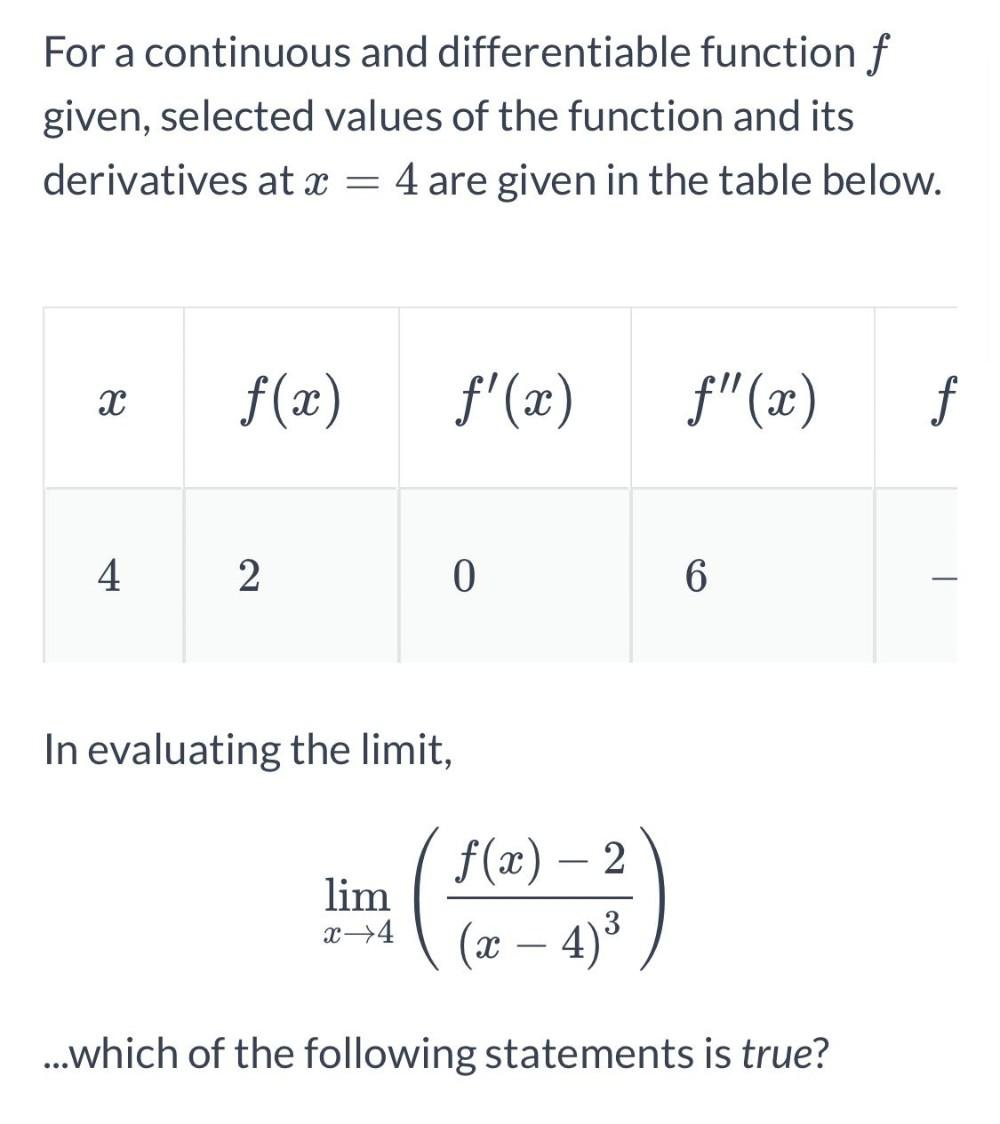Question:

# For a continuous and differentiable function f given, selected values of the function and its derivatives at x = 4 are given inFor a continuous and differentiable function f given, selected values of the function and its derivatives at x = 4 are given in the table below. X X f(x) f'(x) C f"(x) f 4 2 0 6 In evaluating the limit, lim f(x) – 2 (x – 4)3 x +4 3 ...which of the following statements is true?